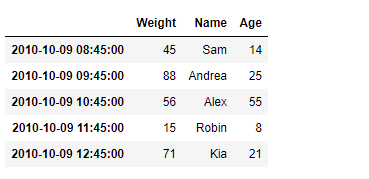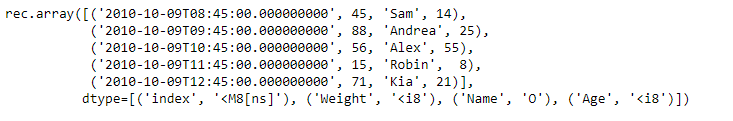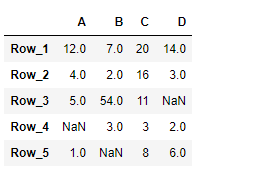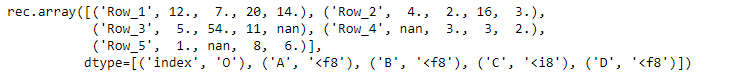# Python | Pandas DataFrame.to_records

Pandas DataFrame is a two-dimensional size-mutable, potentially heterogeneous tabular data structure with labeled axes (rows and columns). Arithmetic operations align on both row and column labels. It can be thought of as a dict-like container for Series objects. This is the primary data structure of the Pandas.

Pandas` DataFrame.to_records()` function convert DataFrame to a NumPy record array. The index will be included as the first field of the record array if requested.

Syntax: DataFrame.to_records(index=True, convert_datetime64=None, column_dtypes=None, index_dtypes=None)

Parameter :
index : bool, default True
convert_datetime64 : Whether to convert the index to datetime.datetime if it is a DatetimeIndex.
column_dtypes : If a string or type, the data type to store all columns
index_dtypes : If a string or type, the data type to store all index levels

Returns : numpy.recarray

Example #1: Use `DataFrame.to_records()` function to convert the given Dataframe to a numpy record array.

 `# importing pandas as pd ` `import` `pandas as pd ` ` `  `# Creating the DataFrame ` `df ``=` `pd.DataFrame({``'Weight'``:[``45``, ``88``, ``56``, ``15``, ``71``], ` `                   ``'Name'``:[``'Sam'``, ``'Andrea'``, ``'Alex'``, ``'Robin'``, ``'Kia'``], ` `                   ``'Age'``:[``14``, ``25``, ``55``, ``8``, ``21``]}) ` ` `  `# Create the index ` `index_ ``=` `pd.date_range(``'2010-10-09 08:45'``, periods ``=` `5``, freq ``=``'H'``) ` ` `  `# Set the index ` `df.index ``=` `index_ ` ` `  `# Print the DataFrame ` `print``(df) `

Output :Now we will use `DataFrame.to_records()` function to convert the given dataframe to a numpy record array representation.

 `# convert to numpy record array ` `result ``=` `df.to_records() ` ` `  `# Print the result ` `print``(result) `

Output :As we can see in the output, the `DataFrame.to_records()` function has successfully converted the given dataframe to a numpy record array representation.

Example #2: Use `DataFrame.to_records()` function to convert the given Dataframe to a numpy record array.

 `# importing pandas as pd ` `import` `pandas as pd ` ` `  `# Creating the DataFrame ` `df ``=` `pd.DataFrame({``"A"``:[``12``, ``4``, ``5``, ``None``, ``1``],  ` `                   ``"B"``:[``7``, ``2``, ``54``, ``3``, ``None``],  ` `                   ``"C"``:[``20``, ``16``, ``11``, ``3``, ``8``],  ` `                   ``"D"``:[``14``, ``3``, ``None``, ``2``, ``6``]})  ` ` `  `# Create the index ` `index_ ``=` `[``'Row_1'``, ``'Row_2'``, ``'Row_3'``, ``'Row_4'``, ``'Row_5'``] ` ` `  `# Set the index ` `df.index ``=` `index_ ` ` `  `# Print the DataFrame ` `print``(df) `

Output :Now we will use `DataFrame.to_records()` function to convert the given dataframe to a numpy record array representation.

 `# convert to numpy record array ` `result ``=` `df.to_records() ` ` `  `# Print the result ` `print``(result) `

Output :As we can see in the output, the `DataFrame.to_records()` function has successfully converted the given dataframe to a numpy record array representation.

My Personal Notes arrow_drop_upCheck out this Author's contributed articles.

If you like GeeksforGeeks and would like to contribute, you can also write an article using contribute.geeksforgeeks.org or mail your article to contribute@geeksforgeeks.org. See your article appearing on the GeeksforGeeks main page and help other Geeks.

Please Improve this article if you find anything incorrect by clicking on the "Improve Article" button below.

Article Tags :

Be the First to upvote.

Please write to us at contribute@geeksforgeeks.org to report any issue with the above content.